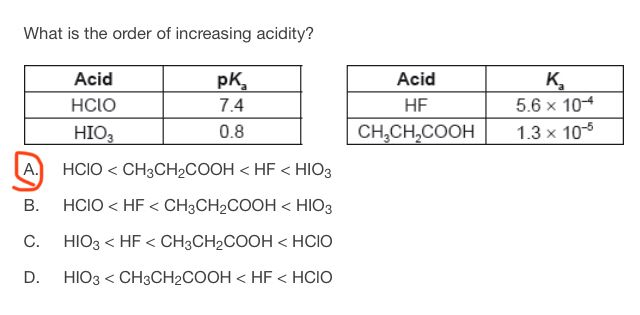# Order of increasing acidityThis is how I thought when I solved this question: the first table has pKa values. So the lower the pKa is, the more stroger is the acid. Thus:
HIO3> HClO

The second table has Ka values. The higher the Ka value is, the more stronger is the acid. Thus: HF> CH3CH2COOH.

When I looked at the different choices, I chose A because it was the only choice which said that HF is stronger than CH3CH2COOH. Have I missed anything in my reasoning?
D. could also be true if first and fourth acids changed places*

The smaller the PKA, the stronger the acid.

This is because of the dissociation rate, with a maximum of 1, and a minimum of 0.

PKA on the other hand is the negative logarithm of KA.

A lower PKA indicated a stronger acid because for a small KA (weak acid) the negative logarithm will be greater.

According to that logic, HICO is the weakest acid, and {HIO_3} is the strongest.

That alone eliminated C&D. The KA of HF is bigger, meaning it is a stronger acid.